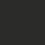# This note has been used to help create the Factors wiki

This is the second note in the series Number Theory: Divisibility. All numbers involved in this note are integers, and letters used in this note stand for integers without further specification.

Recap of Properties:

$(1)$ If $b \mid c,$ and $c \mid a,$ then $b \mid a,$ that is, divisibility is transitive.

$(2)$ If $b \mid a,$ and $b \mid c,$ then $b \mid (a \pm c),$ that is, the set of multiples of an integer is closed under addition and subtraction operations.

By using this property repeatedly, we have, if $b \mid a$ and $b \mid c$, then $b \mid (au+cv)$, for any integers $u$ and $v$. In general, if $a_1,a_2,\cdots,a_n$ are multiples of $b$, then $b \mid (a_1+a_2+\cdots+a_n).$

$(3)$ If $b \mid a$, then $a=0$ or $\lvert a \rvert \geq \lvert b \rvert$. Thus, if $b \mid a$ and $a \mid b$, then $\lvert a \rvert = \lvert b \rvert$.

Clearly, for any two integers $a$ and $b$, $a$ is not always divisible by $b$. But we have the following result, which is called the division algorithm. It is the most important result in elementary number theory.

$(4)$ (The division algorithm) Let $a$ and $b$ be integers, and $b > 0$. Then there is a unique pair of integers $q$ and $r$, such that $a=bq+r \hspace{2mm} \text{and} \hspace{2mm} 0 \leq r < b.$

The integer $q$ is called the (incomplete) quotient when $a$ is divided by $b$, $r$ called the remainder. Note that the values of $r$ has $b$ kinds of possibilities, $0,1,\cdots,b-1$. If $r=0$, then $a$ is divisible by $b$.

It is easy to see that the quotient $q$ in the division algorithm is in fact $\lfloor\frac{a}{b}\rfloor$ (the greatest integer not exceeding $\frac{a}{b}$), and the heart of the division algorithm is the inequality about the remainder $r$: $0 \leq r < b$. We will go back to this point later on.

The basic method of proving $b \mid a$ is to factorize $a$ into the product of $b$ and another integer. Usually, in some basic problems this kind of factorization can be obtained by taking some special value in algebraic factorization equations. The following two factorization formulae are very useful in proving this kind of problems.

$(5)$ If $n$ is a positive integer, then $x^n-y^n=(x-y)(x^{n-1}+x^{n-2}y+\cdots+xy^{n-2}+y^{n-1}).$

$(6)$ If $n$ is a positive odd number, then $x^n+y^n=(x+y)(x^{n-1}-x^{n-2}y+\cdots-xy^{n-2}+y^{n-1}).$

Examples:

Example 1: Prove that $1\underbrace{0\cdots 0}_{200}1$ is divisible by $1001$.

Proof: By $(6)$, we have

$1\underbrace{0\cdots 0}_{200}1=10^{201}+1=(10^3)^{67}+1$

$=(10^3+1)\left[(10^3)^{66}-(10^3)^{65}+\cdots -10^3+1\right]$

$=1001\left[(10^3)^{66}-(10^3)^{65}+\cdots -10^3+1\right].$

Therefore, $1001$ divides $1\underbrace{0\cdots 0}_{200}1$.

Example 2: Let $a$ and $b$ be integers such that $a>b\geq 0$. Show that

$(2^{2^b}+1)\mid (2^{2^a}-1).$

Proof: Take $x=2^{2^{b+1}}$, $y=1$ in $(5)$, and substitute $n$ by $2^{a-b-1}$. We have

$2^{2^a}-1=(2^{2^{b+1}}-1)\left[(2^{2^{b+1}})^{2^{a-b-1}-1}+\cdots+2^{2^{b+1}}+1\right].$

Thus,

$(2^{2^{b+1}}-1)\mid (2^{2^a}-1).$

Since

$2^{2^{b+1}}-1=(2^{2^b}-1)(2^{2^b}+1),$

hence

$(2^{2^b}+1)\mid (2^{2^{b+1}}-1).$

By $(1)$, we have

$(2^{2^b}+1)\mid(2^{2^a}-1),$

and we are done.

Example 3: For a positive integer $n$, let $S(n)$ denote the sum of digits of $n$. Show that $9\mid n$ if and only if $9\mid S(n)$.

Proof: Write $n=a_k\times 10^k +\cdots + a_1\times 10 + a_0$ (where $0\leq a_i \leq 9$, and $a_k \neq 0$), then $S(n)=a_0+a_1+\cdots+a_k$. We have

$n-S(n)=a_k(10^k-1)+\cdots+a_1(10-1)\cdots(1.1)$

For $1 \leq i \leq k$, from $(5)$ we get $9 \mid (10^i-1)$. So every term of the $k$ terms in the RHS of $(1.1)$ is a multiple of $9$, thus $(2)$ implies that their sum is also a multiple of $9$, that is, $9 \mid (n-S(n))$. Hence, the result can be obtained easily.

Example 4: Let $k \geq 1$ be an odd integer. Prove that for any positive integer $n$, $1^k+2^k+\cdots+n^k$ is not divisible by $n+2$.

Proof: When $n=1$ the statement is obviously true. For $n \geq 2$, denote the sum by $A$. Then

$2A=2+(2^k+n^k)+(3^k+(n-1)^k)+\cdots+(n^k+2^k).$

Since $k$ is a positive odd integer, from $(6)$ we know that for every $i \geq 2$, $i^k+(n+2-i)^k$ is divisible by

$i+(n+2-i)=n+2.$

Thus $2A$ has remainder $2$ when divided by $n+2$, which implies that $A$ is not divisible by $n+2$ (note that $n+2>2$).Note by Victor Loh
6 years, 11 months ago

This discussion board is a place to discuss our Daily Challenges and the math and science related to those challenges. Explanations are more than just a solution — they should explain the steps and thinking strategies that you used to obtain the solution. Comments should further the discussion of math and science.

When posting on Brilliant:

• Use the emojis to react to an explanation, whether you're congratulating a job well done , or just really confused .
• Ask specific questions about the challenge or the steps in somebody's explanation. Well-posed questions can add a lot to the discussion, but posting "I don't understand!" doesn't help anyone.
• Try to contribute something new to the discussion, whether it is an extension, generalization or other idea related to the challenge.

MarkdownAppears as
*italics* or _italics_ italics
**bold** or __bold__ bold
- bulleted- list
• bulleted
• list
1. numbered2. list
1. numbered
2. list
Note: you must add a full line of space before and after lists for them to show up correctly
paragraph 1paragraph 2

paragraph 1

paragraph 2

[example link](https://brilliant.org)example link
> This is a quote
This is a quote
    # I indented these lines
# 4 spaces, and now they show
# up as a code block.

print "hello world"
# I indented these lines
# 4 spaces, and now they show
# up as a code block.

print "hello world"
MathAppears as
Remember to wrap math in $$ ... $$ or $ ... $ to ensure proper formatting.
2 \times 3 $2 \times 3$
2^{34} $2^{34}$
a_{i-1} $a_{i-1}$
\frac{2}{3} $\frac{2}{3}$
\sqrt{2} $\sqrt{2}$
\sum_{i=1}^3 $\sum_{i=1}^3$
\sin \theta $\sin \theta$
\boxed{123} $\boxed{123}$